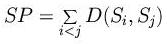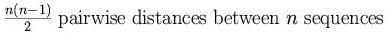4. Dynamic programming

DP for global pairwise alignment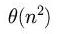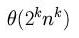n is the length of the sequences and k the number of sequences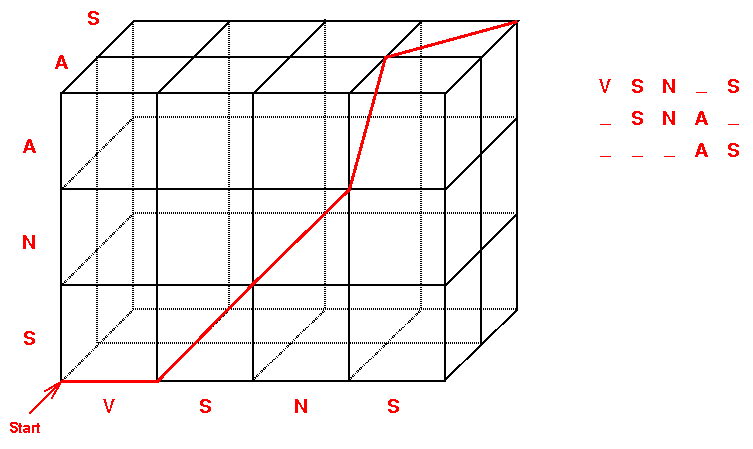Score of a MSA (total distance of a MSA):

• Naive method: For every column in k sequences, there are 2k-1 combinations
• Distance from a consensus
• Tree alignments
• Star alignments
• Sum of pairs (SP-score): The sum of the pairwise score between all pairs of sequences in every column